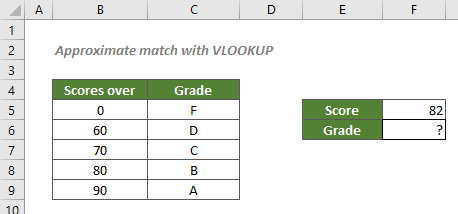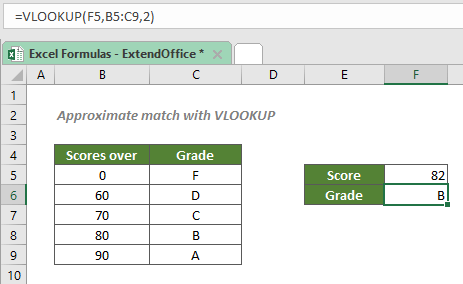## Approximate match with VLOOKUP

To find approximate matches in Excel which can be used to calculate postage based on weight, evaluate employees' performance, calculate income tax based on salary, etc., you can use the VLOOKUP function to retrieve the approximate match as you need.#### How to find approximate matches with VLOOKUP?

To grade a student’s score according to the given table as shown in the screenshot above, you can use the VLOOKUP function. By default, the function performs an approximate match. So, either the function has no range_lookup argument or has TRUE as its range_lookup argument makes no difference. Note that to find approximate matches with VLOOKUP requires the values listed in the first column of the table, i.e., the column where the lookup value is listed, to be sorted in ascending order.

#### Generic syntax

=VLOOKUP(lookup_value,table_array,col_num,range_lookup)

• lookup_value: The value to lookup. Here refers to the given score.
• table_array: The range of cells where to look for approximate matches. Here refers to all the data including the score and grade information.
• col_num: The column in the table_array from which you want to retrieve the matching value.
• range_lookup: TURE or omitted. Forces VLOOKUP to find the first approximate match.

To grade the score of 82, please copy or enter the formula below in the cell F6, and press Enter to get the result:

=VLOOKUP(82,B5:C9,2)

Or, use a cell reference to make the formula dynamic:

=VLOOKUP(F5,B5:C9,2)#### Explanation of the formula

=VLOOKUP(F5,B5:C9,2)

• There is no range_lookup, which indicates an approximate match, and requires the values listed in the first column of the table, i.e., the column B where the lookup value is listed, to be sorted in ascending order.
• VLOOKUP will then find the largest value that is less than or equal to 82, the value in F5, in the range B5:C9. So, it will match the value 80 and lookup the 4th row in the range.
• Then the VLOOKUP function will retrieve the value in the 2nd column of the 4th row, which is B.

#### Related functions

Excel VLOOKUP function

The Excel VLOOKUP function searches for a value by matching on the first column of a table and returns the corresponding value from a certain column in the same row.

#### Related Formulas

Approximate match with INDEX and MATCH

There are times when we need to find approximate matches in Excel to evaluate employees' performance, grade students’ scores, calculate postage based on weight, etc. In this tutorial, we will talk about how to use the INDEX and MATCH functions to retrieve the results we need.

Exact match with VLOOKUP

To find out the information listed in Excel about a specific product, movie or a person, etc., you should take advantage of the VLOOKUP function.

Partial match with VLOOKUP

There are times when you need Excel to retrieve data based on partial information. To solve the problem, you can use a VLOOKUP formula together with wildcard characters - the asterisk (*) and question mark (?).

Left lookup with VLOOKUP

In this tutorial, we will discuss about how to use VLOOKUP to find out the information listed in the left columns in Excel about a given item which is at the right side. We may know that the VLOOKUP function cannot look to its left, so in order to accomplish the task, we will have to use the help of the CHOOSE function.

### The Best Office Productivity Tools

#### Kutools for Excel - Helps You To Stand Out From Crowd

 Popular Features: Find/Highlight/Identify Duplicates  |  Delete Blank Rows  |  Combine Columns or Cells without Losing Data  |  Round without Formula ... Super VLookup: Multiple Criteria  |  Multiple Value   |  Across Multi-Sheets  |  Fuzzy Lookup... Adv. Drop-down List: Easy Drop Down List  |  Dependent Drop Down List  |  Multi-select Drop Down List... Column Manager: Add a Specific Number of Columns  |  Move Columns  |  Unhide Columns  |  Compare Columns to Select Same & Different Cells ... Featured Features: Grid Focus  |  Design View  |  Big Formula Bar  |  Workbook & Sheet Manager  |  Resource Library (Auto Text)  |  Date Picker  |  Combine Worksheets  |  Encrypt/Decrypt Cells  |  Send Emails by List  |  Super Filter  |  Special Filter (filter bold/italic/strikethrough...) ... Top 15 Toolsets:  12 Text Tools (Add Text, Remove Characters ...)  |  50+ Chart Types (Gantt Chart ...)  |  40+ Practical Formulas (Calculate age based on birthday ...)  |  19 Insertion Tools (Insert QR Code, Insert Picture from Path ...)  |  12 Conversion Tools (Numbers to Words, Currency Conversion ...)  |  7 Merge & Split Tools (Advanced Combine Rows, Split Cells ...)  |  ... and more

Kutools for Excel Boasts Over 300 Features, Ensuring That What You Need is Just A Click Away...

Supports Office/Excel 2007-2021 and 365   |   Available in 44 Languages   |   Easy to Uninstall Completely#### Office Tab - Enable Tabbed Reading and Editing in Microsoft Office (include Excel)

• One second to switch between dozens of open documents!
• Reduce hundreds of mouse clicks for you every day, say goodbye to mouse hand.
• Increases your productivity by 50% when viewing and editing multiple documents.
• Brings Efficient Tabs to Office (include Excel), Just Like Chrome, Edge and Firefox.